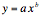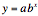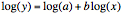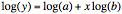Exponentials, logarithms and rescaling of data

In many cases if you were to plot data points obtained from biological measurements (for example mean brain weight as compared to mean total body weight for mammals of various sizes) you would find that the data do not at all fall on a straight line. There are a variety of reasons for this. One example is illustrated by the human auditory system through which we hear sounds. Imagine yourself in a classroom with one other person in it and they drop a pencil (assume the floor doesn't have a rug). You would likely hear the pencil drop. Now imagine the same person dropping the pencil with the classroom full of people talking before class starts. You likely would not hear the pencil fall at all, but it certainly is making the same "sound" (e.g. the physics of the situation hasn't changed). Why does this happen? It is because our hearing is better at detecting "relative" rather than absolute differences between sound levels. Of course this is very simplified since it also depends upon the frequency of the sound, the person’s age, how much exposure they have had to previous loud sound (e.g. rock concerts with too much amplification, having your IPod turned up too loud) but in general much of our perceptual abilities do not occur in a linear manner. Our perceptions are not tuned to detect "additive differences" but rather to detect "multiplicative differences".

Another example is given by population growth. Imagine algae growing in a Petri dish, starting from a single cell. Through time the cell will split (or else die), then each cell will split again then split again, so that the total number of cells in the population of cells in the Petri dish doesn't increase linearly (in an additive manner) through time but multiplicatively (by doubling). If you were to plot the number of cells through time, it would increase geometrically, not linearly.

The above are two examples why exponentials and logarithms are used so much in biology. Exponentials are used to describe something that increases (or decreases) in a multiplicative manner. Logarithms are a way to rescale something that is increasing (or decreasing) in a multiplicative manner so as to measure its increase in a new way that does increase (or decrease) linearly. This arises, as you will recall, from the fact that logarithms turn multiplication into addition  - the logarithm of a product is the sum of the logarithms of the components of the product - log(a*b) = log(a) + lob(b). This would be a good time to refresh your memory of logs and exponentials by reading Sections 5 and 15 of the text.

Allometry

An extremely common relationship that arises over and over again in biology is the notion of an allometric relationship between two measurements. Two variables x and y are said to be allometrically related ifwhere a and b are constants. For a good explanation of allometry you should read through the module BODY SIZE CONSTRAINTS IN XYLEM-SUCKING INSECTS: ALLOMETRIC RELATIONSHIPS posted from the course home page under the Modules section available at

http://www.tiem.utk.edu/~gross/bioed/bealsmodules/allometry.html

and the module on SPECIES-AREA RELATIONSHIPS posted at

http://www.tiem.utk.edu/~gross/bioed/bealsmodules/spec_area.html

Log-log and Semi-log graphs

How do we tell if a non-linear relationship is a better model for how two datasets are related than a linear one? Of course first you should make a scatter plot of the data. If there appears to be a pattern in the data, so that for example one variable tends to increase (or decrease) as the other increases, but a line does not seem to be a good "fit" to the data, then the next step is to try to "transform" the data. In this case, you should try plotting the data on a semi-log or a log-log plot if you have any reason to suspect that the data are related allometrically (use a log-log plot) or if one appears to be geometrically changing with the other (e.g. there is an exponential function describing them such aswhere a and b are constants).

By taking logarithms on both sides of an allometric relationship, you will see that the logarithms of the measurements are then linearly related. To see this, suppose thatThen taking logarithms of both sides giveswhich means that log(y) plotted on the vertical axis versus log(x) plotted n the horizontal axis gives a straight line with slope b and vertical intercept (when log(x) = 0 which occurs when x=1) at log(a). This means that when you plot y vs. x using a log-log plot, you get a straight line.

Similarly, by taking logarithms on both sides of a geometric relationship, you will see that the logarithm of one of the measurements is linearly related to the other measurement. Suppose thatThen taking logarithms of both sides giveswhich implies that log(y) plotted on the vertical axis versus x plotted on the horizontal axis gives a straight line with slope log(b) and vertical intercept (when x = 0) at log(a). This means that when you plot y vs. x using a semi-log plot, you will get a straight line.

Matlab makes it easy to plot these using the functions "semilogy(x,y)" and "loglog(x,y)".